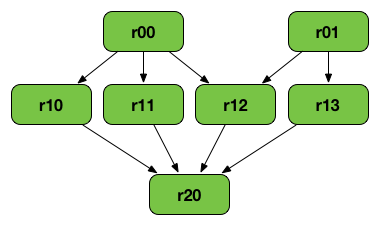# RDD Lineage — Logical Execution Plan¶

RDD Lineage (RDD operator graph or RDD dependency graph) is a graph of all the parent RDDs of an RDD.

RDD lineage is built as a result of applying transformations to an RDD and creates a so-called logical execution plan.

Note

The execution DAG or physical execution plan is the DAG of stages.The above RDD graph could be the result of the following series of transformations:

``````val r00 = sc.parallelize(0 to 9)
val r01 = sc.parallelize(0 to 90 by 10)
val r10 = r00.cartesian(r01)
val r11 = r00.map(n => (n, n))
val r12 = r00.zip(r01)
val r13 = r01.keyBy(_ / 20)
val r20 = Seq(r11, r12, r13).foldLeft(r10)(_ union _)
``````

A RDD lineage graph is hence a graph of what transformations need to be executed after an action has been called.

## Logical Execution Plan¶

Logical Execution Plan starts with the earliest RDDs (those with no dependencies on other RDDs or reference cached data) and ends with the RDD that produces the result of the action that has been called to execute.

Note

A logical plan (a DAG) is materialized and executed when `SparkContext` is requested to run a Spark job.